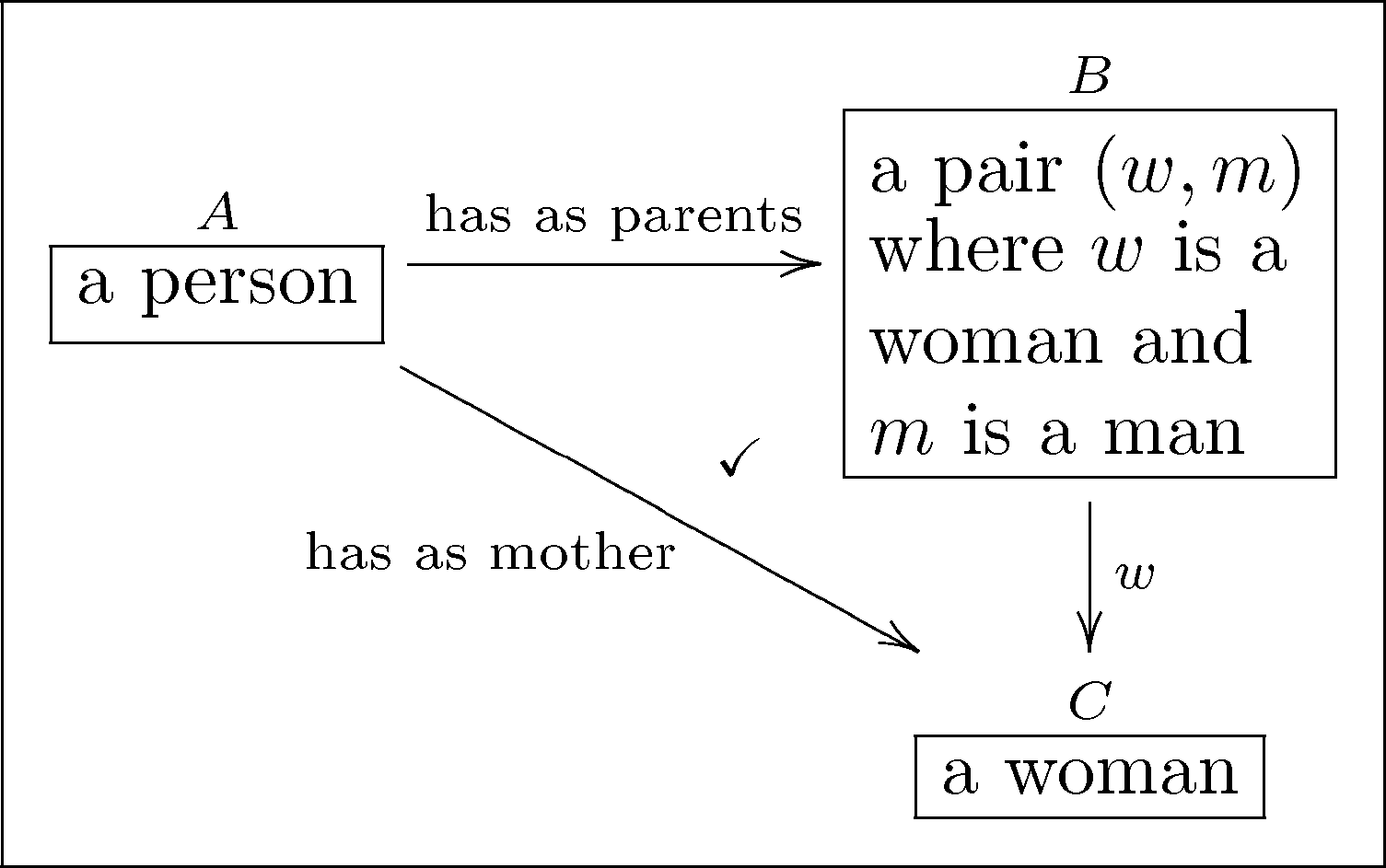## Knowledge Representation in Bicategories of Relations¶

$\quad$$\quad\implies\quad$Evan Patterson
Stanford University, Statistics Department

6th CSLI Workshop on Logic, Rationality, & Intelligent Interaction
June 3, 2017

## Knowledge representation (KR)¶

"An ontology is a specification of a conceptualization."
—Thomas Gruber, co-founder of Siri, Inc.

New applications motivating KR research:

• Semantic Web (RDF, OWL)
• Ontologies in biology and biomedicine (e.g. Gene Ontology)## Description logic (DL)¶

• Dominant formalism for KR today
• Basis for Web Ontology Language (OWL)
• Computationally tractable subset of first-order logic

Example concept constructors: \begin{align} C \sqcap D \qquad&\stackrel{\mathrm{FOL}}{\leadsto}\qquad (C \sqcap D)(x) \leftrightarrow (C(x) \wedge D(x)) \\ \forall R.C \qquad&\stackrel{\mathrm{FOL}}{\leadsto}\qquad (\forall R.C)(x) \leftrightarrow (\forall y. R(x,y) \wedge C(y)) \\ \exists R.\top \qquad&\stackrel{\mathrm{FOL}}{\leadsto}\qquad (\exists R.\top)(x) \leftrightarrow (\exists y. R(x,y)) \end{align}

## Ontology logs (ologs)¶## Relational ologs¶

• Ologs are based on Set, the category of sets and functions
• What about Rel, the category of sets and relations?
• This project: relational ologs, a categorical-relational framework for KR
• Objectives:
1. Understand relationship between logical and algebraic approaches to KR
2. Develop distinctive advantages of categorical KR

## Rel: the category of relations¶

Rel as a monoidal category, via the graphical language of string diagrams

A relation $R: X \to Y$ is a subset of $X \times Y$:

$\quad$### Two basic operations in Rel¶

Composition: Given $R: X \to Y$ and $S: Y \to Z$,

$\quad$$\quad:=\quad \{(x,z): \exists y \in Y. xRy \wedge ySz\}$

Cartesian product: Given $R: X \to Y$ and $S: Z \to W$,

$\quad$$\quad:=\quad \{((x,z),(y,w)): xRy \wedge zSw\}$

### Dagger and diagonals in Rel¶

Dagger: Given $R: X \to Y$,

$\quad$$\quad:=\quad \{(y,x): yRx\}$

Diagonals: For every set $X$,

$\quad$$\quad:=\quad \{(x,(x',x'')): x=x' \wedge x=x''\}$

$\quad$$\quad:=\quad \{(x,*): x \in X\}$

### Examples from description logic¶

Intersection of $R,S: X \to Y$:

$\quad R \sqcap S \quad=\quad$Limited existential quantification of $R:X \to Y$:

$\quad \exists R.\top \quad=\quad$### Rel as a 2-category¶

Rel is a locally posetal 2-category: given relations $R,S: X \to Y$,

$$R \implies S \qquad\text{iff}\qquad R \subseteq S.$$

2-morphisms correspond to subsumption in description logic.

## Abstract categories of relations¶

• Rel cannot stand alone as a KR system
• Need finitary specification language for ontologies
• Two categorical abstractions of relational algebra in literature
1. Allegories (Freyd & Scedrov)
2. Bicategories of relations (Carboni & Walters)

### Bicategories of relations¶

A bicategory of relations is a locally posetal 2-category $\mathcal{B}$ that is also a symmetric monoidal category $(\mathcal{B},\otimes,I)$ with diagonals $(X,\Delta_X,\lozenge_X)_{X \in \mathcal{B}}$ subject to several axioms.

Axioms ensure that

• monoidal product and diagonals behave like the Cartesian product
• 2-morphisms interact properly with other structures

### Relational olog¶

A relational olog is a finitely presented bicategory of relations.

"Finitely presented" means generated by

• a finite set of basic types or object generators
• a finite set of basic relations or morphism generators
• a finite set of subsumbtion axioms or 2-morphisms generators

### Example: Friend of a friend¶

• Basic types: "Person", "Organization"
• Basic relations: "knows", "friend of", "works at", etc.
• Example subsumption axiom

$\quad$$\quad\implies\quad$# Categorical logic¶

• Bicategories of relations are closely related to regular logic
• Regular logic = fragment of typed first-order logic with connectives $\exists, \wedge, \top, =$
• Every regular theory $\mathbb{T}$ has a classifying category $\mathrm{Cl}(\mathbb{T})$, a bicategory of relations
• Every (small) bicategory of relations $\mathcal{B}$ has an internal language $\mathrm{Lang}(\mathcal{B})$, a regular theory

Theorem. For every (small) bicategory of relations $\mathcal{B}$, there is an equivalence of categories $$\mathrm{Cl}(\mathrm{Lang}(\mathcal{B})) \simeq \mathcal{B} \qquad\text{in}\qquad \mathbf{BiRel}.$$